# ISEE Middle Level Math : How to add fractions

## Example Questions

### Example Question #21 : How To Add Fractions

Add: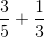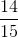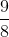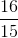Explanation:

Rewrite the fractions with the common denominator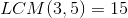, and add numerators: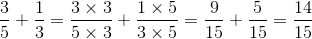### Example Question #22 : How To Add Fractions

Evaluate: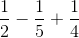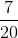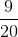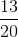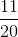Explanation:

The least common denominator of the three fractions is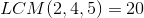, so write each fraction in terms of this denominator: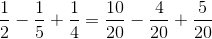By order of operations, subtract, then add: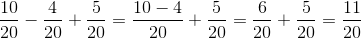,

which is the correct result.

### Example Question #21 : How To Add Fractions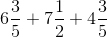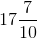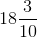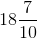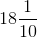Explanation:

Rewrite each fraction with the least common denominator, which is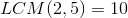: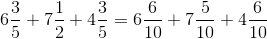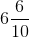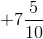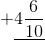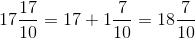### Example Question #681 : Numbers And Operations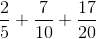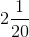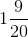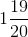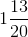Explanation:

Rewrite using the least common denominator, which is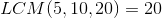; then add numerators: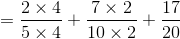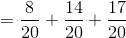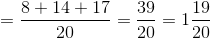### Example Question #31 : How To Add Fractions

Evaluate: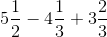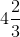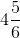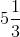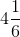Explanation:

Rewrite each as an improper fraction, then as a fraction in terms of the least common denominator, which is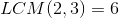: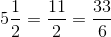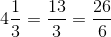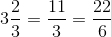Rewrite the expression, evaluate it, and rewrite the result as a mixed number: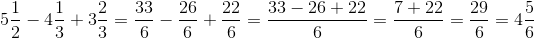### Example Question #32 : How To Add Fractions

Find the sum: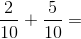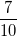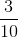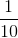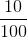Explanation:

Since the denominators of the two fractions are the same, we can simply add the numerators: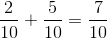### Example Question #411 : Arithmetic

Find the sum: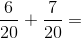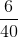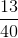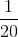Explanation:

Since the denominators of the two fractions are the same, we can simply add the numerators: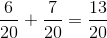### Example Question #34 : How To Add Fractions

Find the sum: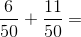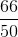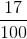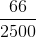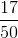Explanation:

Since the denominators of the two fractions are the same, we can simply add the numerators: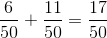### Example Question #35 : How To Add Fractions

Solve: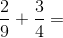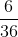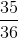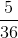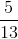Explanation:

Find the least common denominator betweenand. In this case, it is.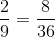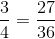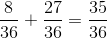### Example Question #36 : How To Add Fractions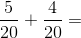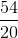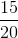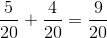Answer: